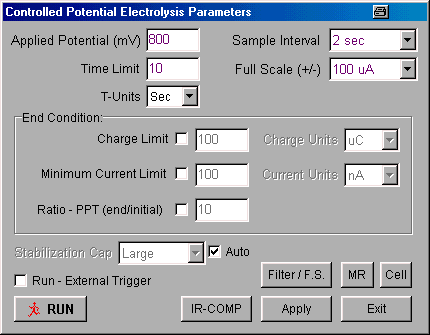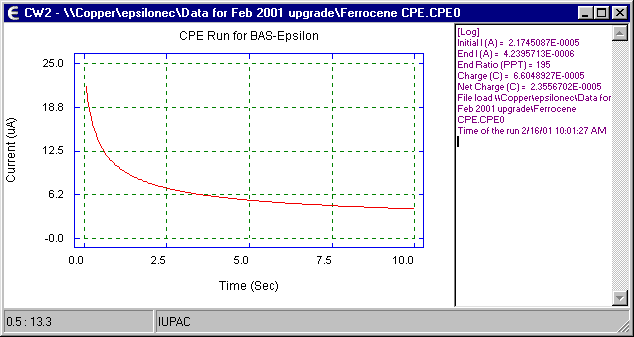# Controlled Potential Electrolysis

#### Introduction

The principle behind the Controlled Potential Electrolysis (CPE) experiment is very simple. If only the oxidized species is initially present, then the potential is set at a constant value sufficiently negative to cause rapid reduction and is maintained at this value until only the reduced species is present in solution. The total charge passed during the CPE experiment (Q) is calculated by integrating the current and is related to the number of electrons transferred per molecule (n) and the number of moles of the oxidized species initially present (N) through Faraday's law:

Q = nFN

where F is Faraday's constant (96500 C mol-1). Therefore, if one of n or N is known, the other can be calculated. Hence, CPE has both analytical and synthetic applications, and is a standard technique on the epsilon.

#### Setting Up a Constant Potential Electrolysis Experiment

The parameter values for CPE are set using the Change Parameters dialog box (Fig1) in either the Experiment menu or the pop-up menu.Figure 1. Change Parameters dialog box for controlled potential eletrolysis.

1. Potential values are entered in mV, and time values are entered in minutes or seconds (selected using T-Units)
2. The time resolution of the data is specified by the Sample Interval (e.g., the default condition is that a data point is recorded every second).
3. There are two gain stages for the current-to-voltage converter. The default values of these stages that are used for a given current Full Scale value are determined by the software. However, they can be adjusted manually using the Filter / F.S. dialog box. This dialog box is also used to change the analog Noise Filter Value settings from the default values set by the software.
4. A stabilizing capacitor (Stabilization Cap) between the auxiliary and reference electrodes is switched in during the CPE experiment. The Auto default capacitor (Large - 0.1 uF) can be manually changed to Small (0.01 uF) or No Cap (no capacitor).
5. The default condition of the cell is that the cell is On (i.e., the electronics are connected to the electrodes) during the experiment, and is Offbetween experiments. However, the potential can be switched Onbetween experiments using the Cell dialog box. HOWEVER, THIS OPTION SHOULD BE USED WITH CAUTION SINCE CONNECTING OR DISCONNECTING THE ELECTRODES WHEN THE CELL IS ON CAN RESULT IN DAMAGE TO THE POTENTIOSTAT, THE CELL, AND/OR THE USER!
6. A series of identical experiments on the same cell can be programmed using the MR (Multi-Run) option. However, if any of the optional End Conditions are checked, any MR settings are ignored.
7. Clicking Exit will exit the dialog box without saving any changes made to the parameter values. Any changes can be saved by clicking Apply before exiting.
8. The end of the CPE experiment can be set by the user in a number of ways. The most basic criterion is the Time Limit, which must be set by the user; that is, the experiment will end after a user-defined time period. However, there are three optional criteria that can also be set:
• Charge Limit - The absolute value of the charge limit should be specified
• Minimum Current Limit - the absolute minimum current value should be specified
• Ratio - PPT (end/initial) - the criterion is the ratio of the final current to the initial current in parts per thousand
Any one of these three optional criteria can be used in addition to the Time Limit. However, it is important to note that the Time Limit always takes precedence; that is, if the Time Limit is attained before e.g., the charge exceeds the Charge Limit, the experiment will end. It should also be noted that there will typically be a delay of 1 or 2 seconds between the time the selected criterion is exceeded and the termination of the experiment, due to the time required for data processing. If any of these optional criteria are used, the multi-run capability is disabled.
9. Range of allowed parameter values:
• Potential = -3275 - +3275 mV
• Sample Interval = 0.05, 0.1, 0.2, 0.5, 1, 2, 5, 10, 30 and 60 sec
• Time Limit = 0 - 32000 in sec or min (the maximum value allowed for the Time Limit is also determined by the Sample Interval, since a maximum of 64000 data points can be recorded in one experiment (e.g, the shorter the Sample Interval, the shorter the experiment)).
10. Once the parameters have been set, the experiment can be started by clicking Run (either in this dialog box, in the Experiment menu, in the pop-up menu, on the Tool Bar, or using the F5 key).
11. Neither the Pre Run Drops function nor the internal dummy cell are available for CPE.

The potential required for a CPE experiment is determined by the redox potential of the analyte (measured by e.g., cyclic voltammetry). For a reduction, the ideal potential is ca. 200 mV more negative than the redox potential so that the rate of electrolysis is controlled by the rate of mass transport to the working electrode. However, it is not always possible to use a potential too far removed from the redox potential due to electrolysis of other electroactive materials (e.g., electrolyte, solvent ,or other components of the solution mixture).

The cell required for CPE is significantly different to that required for voltammetry experiments (in which only a very small fraction of the electroactive molecule of interest is electrolyzed). The rate of electrolysis is enhanced by using a working electrode with a large surface area (e.g., platinum gauze, reticulated vitreous carbon or a mercury pool) and an auxiliary electrode with a large surface area (e.g., platinum coil or gauze); in addition, the solution is stirred to increase the rate of mass transport to and from the working electrode. The auxiliary electrode must be isolated from the working electrode to prevent species that are electrogenerated at the auxiliary electrode from interfering with electrolysis at the working electrode. However, care must be taken when choosing the material used to isolate the auxiliary electrode from the working electrode, since high resistance material may affect the efficiency of the electrolysis.

The output from a CPE experiment is a current vs. time plot (a typical example is shown in Fig2).Figure 2. Current vs. time plot for constant potential electrolysis.

The following parameters are displayed in a separate window during the experiment, and are updated every second:

• Elapsed Time
• Initial Current (the current at the start of the experiment)
• Current (the most recent current value)
• Current Ratio (ratio of the most recent current value to the initial current value in part per thousand)
• Charge (the accumulated charge)

At the end of the experiment, the following parameters are displayed in the Text Info box to the right of the display (Fig2):

• Initial Current I
• End Current I (the current value at the end of the experiment, calculated as the average of the last 5 current values)
• End Current Ratio
• Charge
• Net Charge

Net Charge is calculated by subtracting the background charge from the total charge passed during the experiment (Charge). The background current is assumed to be constant during the experiment, and equal to End I. Therefore, the background charge is equal to the product of the background current and the experimental time. Once the Net Charge has been calculated, it can be used to calculate the number of electrons transferred or the amount of material electrolyzed (in moles) through Faraday's Law:

Q = nFN

 where: n = number of electrons transferred/molecule F = Faraday's constant (96,485 C mol-1) N = amount of material electrolyzed (mol)